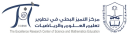# Area Model IntroductionDownloadEmbed close Embed a running copy of this simulation Use this HTML to embed a running copy of this simulation. You can change the width and height of the embedded simulation by changing the "width" and "height" attributes in the HTML. Embed an image that will launch the simulation when clicked
Click to Run
Use this HTML code to display a screenshot with the words "Click to Run". Factors Products Area Model PhET is supported byand educators like you.

### Topics

• Factors
• Products
• Area Model
• Multiplication
• Partial Products

### Description

Build rectangles of various sizes and relate multiplication to area. Partition a rectangle into two areas to discover the distributive property.

### Sample Learning Goals

• Recognize that area represents the product of two numbers.
• Develop and justify a strategy that uses the area model to simplify a multiplication problem.
• Represent a multiplication problem as the proportional area of a rectangle.
• Looks for patterns in the total area calculation.

### Standards Alignment

#### Common Core - Math

2.NBT.A.2
Count within 1000; skip-count by 5s, 10s, and 100s.
2.OA.C.4
Use addition to find the total number of objects arranged in rectangular arrays with up to 5 rows and up to 5 columns; write an equation to express the total as a sum of equal addends.
3.MD.C.7
Relate area to the operations of multiplication and addition.
3.MD.C.7c
Use tiling to show in a concrete case that the area of a rectangle with whole-number side lengths a and b + c is the sum of a × b and a × c. Use area models to represent the distributive property in mathematical reasoning.
3.MD.C.7d
Recognize area as additive. Find areas of rectilinear figures by decomposing them into non-overlapping rectangles and adding the areas of the non-overlapping parts, applying this technique to solve real world problems.
3.OA.A.1
Interpret products of whole numbers, e.g., interpret 5 × 7 as the total number of objects in 5 groups of 7 objects each. For example, describe a context in which a total number of objects can be expressed as 5 × 7.
3.OA.A.3
Use multiplication and division within 100 to solve word problems in situations involving equal groups, arrays, and measurement quantities, e.g., by using drawings and equations with a symbol for the unknown number to represent the problem.1
3.OA.B.5
Apply properties of operations as strategies to multiply and divide.2 Examples: If 6 × 4 = 24 is known, then 4 × 6 = 24 is also known. (Commutative property of multiplication.) 3 × 5 × 2 can be found by 3 × 5 = 15, then 15 × 2 = 30, or by 5 × 2 = 10, then 3 × 10 = 30. (Associative property of multiplication.) Knowing that 8 × 5 = 40 and 8 × 2 = 16, one can find 8 × 7 as 8 × (5 + 2) = (8 × 5) + (8 × 2) = 40 + 16 = 56. (Distributive property.)
4.OA.A.1
Interpret a multiplication equation as a comparison, e.g., interpret 35 = 5 × 7 as a statement that 35 is 5 times as many as 7 and 7 times as many as 5. Represent verbal statements of multiplicative comparisons as multiplication equations.
Version 1.2.1

### Teacher-Submitted Activities

Middle School Math Sim AlignmentAmanda McGarry MS Other Mathematics
Area Model Intro Simulation Nailah Shah-Taylor K-5 Discuss
Guided
Mathematics
PRIMARIA: Alineación con programas de la SEP México (2011 y 2017)Diana López MS
K-5
Demo
Guided
Lab
HW
Discuss
Physics
Mathematics
Astronomy
Chemistry
Albanian All shqipModeli i Syprinës: Hyrje
Arabic All العربيةمقدمة مساحة النموذج
Basque All EuskaraAzalera-modelorako sarrera
Bosnian All BosanskiMatematički model površina - Uvod
Chinese (Simplified) All 中文 (中国)面积模型入门
Chinese (Traditional) All 中文 (台灣)Area Model Introduction_面積模型介紹
Danish All DanskArealmodel, introduktion
Dutch All NederlandsOppervlakte model inleiding
Finnish All suomiPinta-alamallin johdanto
French All françaisIntroduction à la modélisation par les aires
German All DeutschFlächenmodell Einführung
Greek All ΕλληνικάΥπολογισμός εμβαδού - Εισαγωγή
Gujarati All Gujaratiક્ષેત્રફળના મોડેલની પ્રસ્તાવના - નિકુંજ સવાણી દ્વારા
Hindi All हिंदीनमूने का क्षेत्रफल -परिचय
Hungarian All magyarTerületmodell: bevezetés
Italian All italianoRappresentazione grafica della moltiplicazione: introduzione
Japanese All 日本語面積の基本
Kazakh All KazakhАуданын табудың негіздері
Korean All 한국어면적 모형: 소개
Latvian All LatviešuLauka modeļa ievads
Mongolian All Монгол (Монгол)Талбайн Загварын Танилцуулга
Persian All فارسیمعرفی مدل مساحت
Polish All polskiModel pola - wstęp
Portuguese All portuguêsModelo de Área: Introdução
Portuguese (Brazil) All português (Brasil)Modelo de Área: Intro
Russian All русскийОсновы нахождения площади
Serbian All СрпскиМатематика преко површина-Увод
Sinhalese All Sinhaleseවර්ගඵලය ආකෘති හඳුන්වාදීම
Spanish All españolModelo de Áreas: Introducción
Spanish (Mexico) All español (México)Modelo de Áreas: Introducción
Spanish (Peru) All español (Perú)Modelo de Área. Introducción
Swedish All svenskaAreamodellen -Introduktion
Turkish All TürkçeAlan Modeline Giriş
Ukrainian All українськаПлощинна модель. Вступ
Vietnamese All Tiếng ViệtGiói thiệu Mô hình diện tích

HTML5 sims can run on iPads and Chromebooks, as well as PC, Mac, and Linux systems.

iOS 12+ Safari

Android:
Not officially supported. If you are using the HTML5 sims on Android, we recommend using the latest version of Google Chrome.

Chromebook:
The HTML5 and Flash PhET sims are supported on all Chromebooks.
Chromebook compatible sims

Windows Systems:

Macintosh Systems:

Linux Systems:

Design Team Third-party Libraries Thanks To
• Jonathan Olson (developer)
• Karina Hensberry
• Susan Miller
• Ariel Paul
• Kathy Perkins
• Mariah Hermsmeyer (artwork)
• Diana López Tavares (artwork)
• almond-0.2.9.js
• base64-js-1.2.0.js
• FileSaver-b8054a2.js
• font-awesome-4.5.0
• game-up-camera-1.0.0.js
• he-1.1.1.js
• himalaya-0.2.7.js
• jama-1.0.2
• jquery-2.1.0.js
• lodash-4.17.4.js
• pegjs-0.7.0.js
• seedrandom-2.4.2.js
• text-2.0.12.js
• TextEncoderLite-3c9f6f0.js